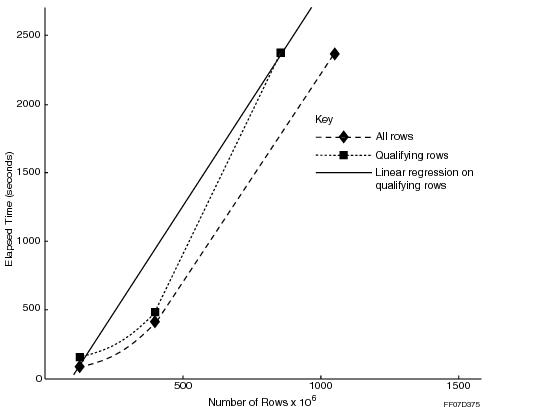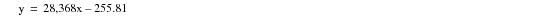# 15.00 - Cost of Join Index Creation - Teradata Database

prodname
vrm_release
15.00
category
User Guide
featnum
B035-1094-015K

### Cost of Join Index Creation

The cost of creating a join index is described in terms of time: how long does it take to create the index? Equally important in many situations is the question of how many times a query for which a join index was designed must be performed to offset the creation time by recovering response time.

Different types of join index require a longer or shorter time to create.

If your processing environment requires that join indexes be created and dropped on a regular basis, the cost of creating the index becomes a critical factor that must be considered carefully in determining its worth.

Because of this creation overhead, you should evaluate the benefit of a join index created to enhance your standard queries vis‑a-vis its creation cost if you must drop and create your join indexes frequently. If the gross effect of an index is negative with respect to overall system performance, you might not want to create it even if it enhances the performance of particular queries.

Unfortunately, it is not possible to predict join index creation times with any degree of accuracy because there are too many significant variables involved to be able to produce a useful predictive model. This multivariate complexity also prevents the derivation of a useful predictive model for maintenance overhead or even for query time reductions.

#### Example

Consider the following example. Empirical testing indicates that one of the most simple relationships is that between the time required to create an in-place aggregate join index and the number of rows in the base tables. Even this simple relationship is complicated by the fact that the resulting creation time is likely to be more closely related to the number of rows that qualify for the join rather than the total number of rows.

The following table indicates a measured relationship between the elapsed creation time for a join index and the number of qualified rows.

 Elapsed Time (seconds) Number of Qualified Rows x 106 133 90 415 300 2,344 900

These numbers, along with the total number of rows in each test and a least squares linear regression of number of qualifying rows on elapsed time, are graphed in the following figure:The equation for this regression is as follows:This translates into physical terms as follows:where the value for Create time is expressed in elapsed seconds and the order of magnitude for Number of qualified rows is expressed in millions of rows.

The computed coefficient of determination for this regression, R2, is 0.9819. This means that 98% of the variance in the sample data is accounted for by this equation, for which the correlation coefficient is 0.991. In other words, the line is nearly a perfect fit to the data.

A sanity check of the data reveals that this regression might not always be as accurate as it seems to be upon initial scrutiny. For example, consider the 300 million row case. The measured elapsed time value is 415 seconds, but the predicted value is 595.23 seconds, a difference of just over 180 seconds, which is an error of 43%.

There are several possible explanations for this discrepancy, but the message to be taken away from this example is that while the model performs well in predicting the general direction of how much time it should take to create a join index with a given number of qualifying rows, the absolute accuracy of the prediction might vary considerably at some points on the curve.

#### Creation and Elapsed Query Times for Different Join Indexes

The following tables list the creation and elapsed query times for several different kinds of join indexes.

Note that when the definition of a join index can be covered by an existing join index, the existing index can be used to create the join index.

In the following table, when calculating the values for the Query Ratio and Benefit columns, query times of less than 1 second were rounded up to 1.0 seconds.

 Approximate Creation Costs and Query Benefits in Terms of Elapsed Query Time Join Index Type Creation Cost (seconds) Elapsed Query Time (seconds) Query Ratio (seconds) Benefit (seconds) Benefit Percent Without Join Index With Join Index Single-table aggregate 51 41 < 1 41.00 40 98 2-table in-place aggregate 135 107 < 1 107.00 106 99 4-table in-place aggregate 279 243 < 1 243.00 242 100 Single-table simple 199 80 63 1.27 17 21 2-table in-place 190 116 78 1.49 38 33 2-table in-place outer join 231 147 98 1.50 49 33 2-table foreign aggregate 232 230 < 1 230.00 229 100 2-table ad hoc aggregate 397 357 < 1 357.00 356 100

In the following table, the figure <<1 means the CPU path per row time was too short to measure. When calculating the values for the Benefit column, query times of less than 1 second were rounded up to 1.0 seconds. The Benefit value is based on elapsed query time rather than CPU path per row time.

 Creation Costs and Query Benefits in Terms of CPU Path Per Row Join Index Type Creation Cost (μseconds) Query Time CPU Path Per Row (μseconds) Benefit Benefit Percentage Without Join Index With Join Index Single-table aggregate 9 7 << 1 40 98 2-table in-place aggregate 7 6 << 1 106 99 4-table in-place aggregate 10 8 << 1 242 100 Single-table simple 33 14 11 17 21 2-table in-place 14 10 7 38 33 2-table in-place outer join 16 11 7 49 33 2-table foreign aggregate 19 19 << 1 229 100 2-table ad hoc aggregate 34 30 << 1 356 100

You can use these figures, which are derived from tests on a two node system, to scale an approximately linear adjustment for your own table sizes and configuration.